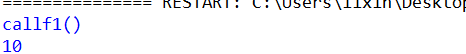什么是装饰器？

def f1(x):     return x*2
def f2(x):     return x*x
def f3(x):     return x*x*x

def f1(x):    print 'call f1()' return x*2
def f2(x):    print 'call f2()' return x*x
def f3(x):    print 'call f3()' return x*x*x

(1)、可以接受函数作为参数

(2)、可以返回函数

(3)、那么可否通过接收一个函数，内部对其包装，然后返回一个新函数，这样子动态的增强函数功能

def f1(x): return x*2

def new_fn(f):
'''装饰器函数'''
def fn(x):
print 'call' + f.__name__ + '()'
return f(x)
return fn

g1 = new_fn(f1)
print g1(5)通过高阶函数达到我们的目的。

def f1(x): return x*2

def new_fn(f):
'''装饰器函数'''
def fn(x):
print 'call' + f.__name__ + '()'
return f(x)
return fn

f1 = new_fn(f1)
print f1(5)是一样的。但是这样子的话，f1的原始定义函数就被彻底隐藏了。

(1)、python内置的 @ 语法就是为了简化装饰器的使用。

代码一：
@new_fn
def f1(x): return x*2

print f1(5)
代码二：
def f1(x): return x*2

f1 = new_fn(f1)
print f1(5)是一样的。

(1)、打印日志：@log    (2)、检测性能：@performance

(3)、数据库事务：@transaction   (4)、URL路由：@post('/register')

(1)、定义自己先要执行的函数

(2)、

def new_fn(要执行的函数名字):
def fn(要执行的参数函数参数/或者没有参数)：
添加要添加的函数功能
return f(x)  函数的执行结果
return fn

(3)、装饰器进行修饰